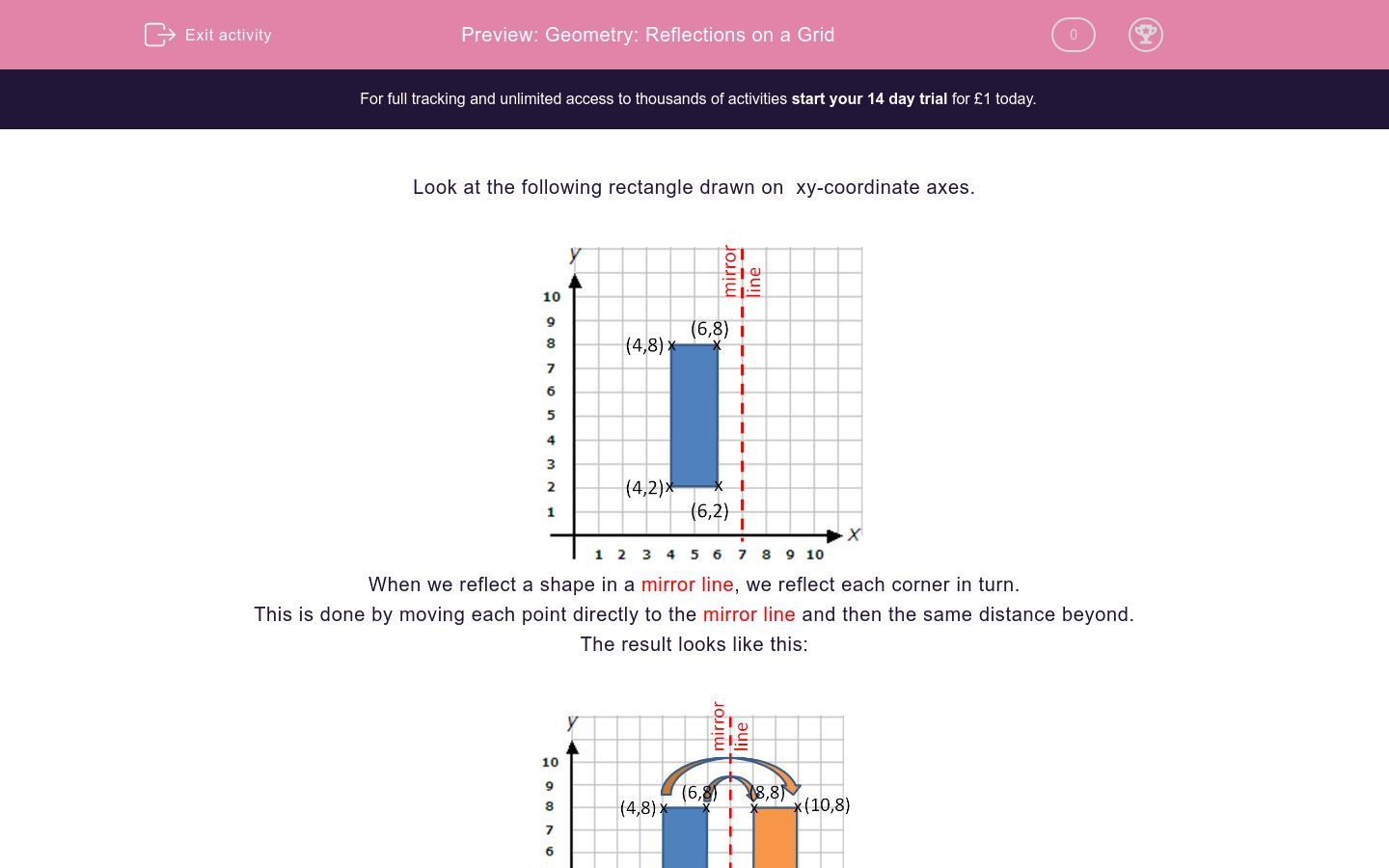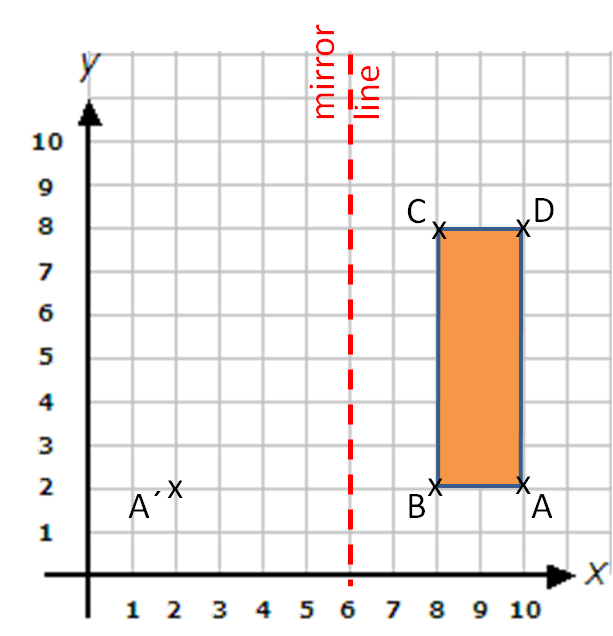# Geometry: Reflections on a Grid

In this worksheet, students reflect points and shapes in the given mirror line on a coordinate grid.Key stage:  KS 2

Curriculum topic:   Maths and Numerical Reasoning

Curriculum subtopic:   Transformations

Difficulty level:### QUESTION 1 of 10

Look at the following rectangle drawn on  xy-coordinate axes.When we reflect a shape in a mirror line, we reflect each corner in turn.

This is done by moving each point directly to the mirror line and then the same distance beyond.

The result looks like this:When you are writing the co-ordinates, you must always remember to put the brackets around them.

Look at this diagram, which shows a rectangle ABCD, which is to be reflected in the mirror line to give rectangle A'B'C'D'.

The point A has been reflected in the mirror line to the point A'.

Write down the coordinates of B'. (Don't forget the brackets!)Look at this diagram, which shows a rectangle ABCD, which is to be reflected in the mirror line to give rectangle A'B'C'D'.

The point A has been reflected in the mirror line to the point A'.

Write down the coordinates of C'. (Don't forget the brackets!)Look at this diagram, which shows a rectangle ABCD, which is to be reflected in the mirror line to give rectangle A'B'C'D'.

The point A has been reflected in the mirror line to the point A'.

Write down the coordinates of D'. (Don't forget the brackets!)Look at this diagram, which shows a rectangle ABCD, which is to be reflected in the mirror line to give rectangle A'B'C'D'.

Write down the coordinates of A'.Look at this diagram, which shows a rectangle ABCD, which is to be reflected in the mirror line to give rectangle A'B'C'D'.

Write down the coordinates of B'.Look at this diagram, which shows a rectangle ABCD, which is to be reflected in the mirror line to give rectangle A'B'C'D'.

Write down the coordinates of C'.Look at this diagram, which shows a rectangle ABCD, which is to be reflected in the mirror line to give rectangle A'B'C'D'.

Write down the coordinates of D'.Look at this diagram, which shows a rectangle ABCD, which is to be reflected in the mirror line to give rectangle A'B'C'D'.

The point B has been reflected in the mirror line to the point B'.  Notice that we count 3 diagonals from B as we move to this mirror line and then 3 diagonals beyond to get to B'.

Write down the coordinates of C'.Look at this diagram, which shows a rectangle ABCD, which is to be reflected in the mirror line to give rectangle A'B'C'D'.

The point B has been reflected in the mirror line to the point B'.  Notice that we count 3 diagonals from B as we move to this mirror line and then 3 diagonals beyond to get to B'.

Write down the coordinates of D'.Look at this diagram, which shows a rectangle ABCD, which is to be reflected in the mirror line to give rectangle A'B'C'D'.

The point B has been reflected in the mirror line to the point B'.  Notice that we count 3 diagonals from B as we move to this mirror line and then 3 diagonals beyond to get to B'.

Write down the coordinates of A'.• Question 1

Look at this diagram, which shows a rectangle ABCD, which is to be reflected in the mirror line to give rectangle A'B'C'D'.

The point A has been reflected in the mirror line to the point A'.

Write down the coordinates of B'. (Don't forget the brackets!)(4,2)
(4, 2)
• Question 2

Look at this diagram, which shows a rectangle ABCD, which is to be reflected in the mirror line to give rectangle A'B'C'D'.

The point A has been reflected in the mirror line to the point A'.

Write down the coordinates of C'. (Don't forget the brackets!)(4,8)
(4, 8)
• Question 3

Look at this diagram, which shows a rectangle ABCD, which is to be reflected in the mirror line to give rectangle A'B'C'D'.

The point A has been reflected in the mirror line to the point A'.

Write down the coordinates of D'. (Don't forget the brackets!)(2,8)
(2, 8)
• Question 4

Look at this diagram, which shows a rectangle ABCD, which is to be reflected in the mirror line to give rectangle A'B'C'D'.

Write down the coordinates of A'.(10,10)
(10, 10)
• Question 5

Look at this diagram, which shows a rectangle ABCD, which is to be reflected in the mirror line to give rectangle A'B'C'D'.

Write down the coordinates of B'.(8,10)
(8, 10)
• Question 6

Look at this diagram, which shows a rectangle ABCD, which is to be reflected in the mirror line to give rectangle A'B'C'D'.

Write down the coordinates of C'.(8,7)
(8, 7)
• Question 7

Look at this diagram, which shows a rectangle ABCD, which is to be reflected in the mirror line to give rectangle A'B'C'D'.

Write down the coordinates of D'.(10,7)
(10, 7)
• Question 8

Look at this diagram, which shows a rectangle ABCD, which is to be reflected in the mirror line to give rectangle A'B'C'D'.

The point B has been reflected in the mirror line to the point B'.  Notice that we count 3 diagonals from B as we move to this mirror line and then 3 diagonals beyond to get to B'.

Write down the coordinates of C'.(5,8)
(5, 8)
• Question 9

Look at this diagram, which shows a rectangle ABCD, which is to be reflected in the mirror line to give rectangle A'B'C'D'.

The point B has been reflected in the mirror line to the point B'.  Notice that we count 3 diagonals from B as we move to this mirror line and then 3 diagonals beyond to get to B'.

Write down the coordinates of D'.(5,10)
(5, 10)
• Question 10

Look at this diagram, which shows a rectangle ABCD, which is to be reflected in the mirror line to give rectangle A'B'C'D'.

The point B has been reflected in the mirror line to the point B'.  Notice that we count 3 diagonals from B as we move to this mirror line and then 3 diagonals beyond to get to B'.

Write down the coordinates of A'.(2,10)
(2, 10)
---- OR ----

Sign up for a £1 trial so you can track and measure your child's progress on this activity.

### What is EdPlace?

We're your National Curriculum aligned online education content provider helping each child succeed in English, maths and science from year 1 to GCSE. With an EdPlace account you’ll be able to track and measure progress, helping each child achieve their best. We build confidence and attainment by personalising each child’s learning at a level that suits them.

Get started